# Algebra - math word problems

#### Number of problems found: 3225

• The rectangularThe rectangular trapezoid has bases 15 dm and 8 dm long and the length of the inclined arm is 12 dm. How long is the other arm of the trapezoid?
• Parallelogram ABCDWe have the parallelogram ABCD, where AB is 6.2 cm BC is 5.4 cm AC is 4.8 cm calculate the height on the AB side and the angle DAB
• SelectSelect the statement that describes this expression: 5 x (3 + 4) - 4. a) 5 times the sum of 3 and 4, then subtract 4 b) 5 times 3 plus 4 minus 4 c) 5 more than the sum of 3 and 4, and then subtract 4 d) 5 times 3 plus the difference of 3 and 4 What is the
• Scrapbook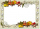Jo is making a scrapbook for her best friend’s 5th grade graduation. She used 72 photos with 6 photos on each page. Jo had to remove two of the photos on each page in order to make room for the writing she wanted to include. Write an expression represents
• Isosceles trapezoidFind the height in an isosceles trapezoid if the area is 520 cm2 and the base a = 25 cm and c = 14 cm. Calculate the interior angles of the trapezoid.
• Part-timers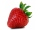Six part-timers would take seven hours to pick strawberries. How long would this work take if two more temporary workers were added after three hours?
• Diamond and anglesFind the area of a diamond with a side of 5 cm if you know that the internal angles in the diamond are 60° and 120°.
• Retirement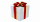To purchase a gift to a retiring co-worker, everyone had to pay EUR 15. Because 4 workers were ill on the day the money was collected, everyone present had to pay additional 1 euro except retiring one. How many workers did the workshop have and how much d
• Three subjects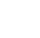In a class of 40 students, 18 passed mathematics, 19 passed accounts, 16 passed economics, 5 mathematics and accounts only, 6 mathematics only, 9 accounts only, 2 accounts and economics only, if each student offered at least one of the subjects. a) how ma
• Two workers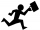One worker makes a part 4 hours and the second 9 hours later than they would make the same part together. How long does it take for each worker to make the part himself?
• Above water surface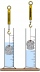If we remove the stone from the water, we apply a force of 120N. How much force will we have to exert if we move the stone above the water surface? The density of the stone is 5000 kg/m ^ 3.
• The surfaceThe surface of the cylinder is 1570 cm2, its height is 15 cm. Find its volume and radius of the base.
• Evaluate 9Evaluate expression: 3 { 4 − 4 ( 5 − 12 ) } + 20
• Parallelogram diagonalsFind the area of a parallelogram if the diagonals u1 = 15 cm, u2 = 12 cm and the angle formed by them is 30 degrees.
• Poisson distribution - daisies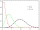The meadow behind FLD was divided into 100 equally large parts. Subsequently, it was found that there were no daisies in ten of these parts. Estimate the total number of daisies in the meadow. Assume that daisies are randomly distributed in the meadow.
• Edges of the cuboid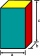Find the length of the edges of the cuboid, which has the following dimensions: width is 0.4 m; the height is 5.8 dm and the block can hold 81.2 liters of fluid.
• Chess piecesThere are 5 black chess pieces in the box. How many white pieces should we add to this box so that the probability of pulling out a black piece is 1: 4?
• Hour salary + fix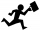Devin recently hired a contractor to do some necessary work. The contractor gave a quote of \$395 for parts plus \$62 an hour for labor. Let x  represent the number of labor hours worked. Write an algebraic expression to represent the total cost for the rep
• The profitThe profit made by a business in 2018 was triple that made in 2019. The profit made in 2020 was R 3 million less than the profit made in 2019. If the total profit in these 3 years was R 67 million, what was the profit made in 2020?
• Center of gravity and medianIn the isosceles triangle ABC, the center of gravity T is 2 cm from the base AB. The median parallel to the AB side measures 4 cm. What is the area of the ABC triangle?

Do you have an interesting mathematical word problem that you can't solve it? Submit a math problem, and we can try to solve it.

We will send a solution to your e-mail address. Solved examples are also published here. Please enter the e-mail correctly and check whether you don't have a full mailbox.

Please do not submit problems from current active competitions such as Mathematical Olympiad, correspondence seminars etc...